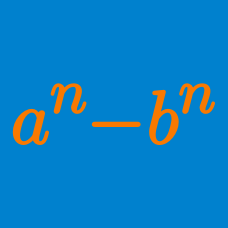Algebra

# Factorization of Rational Functions

If $P(n)=\frac{n^3+n^2-2n}{n^2-4}+\frac{n+1}{2+n-n^2}$, what is the value of $\lfloor P(409) \rfloor + \lfloor P(423) \rfloor$?

Details and assumptions

The function $\lfloor x \rfloor: \mathbb{R} \rightarrow \mathbb{Z}$ refers to the greatest integer smaller than or equal to $x$. For example, $\lfloor 2.3 \rfloor = 2$ and $\lfloor -5 \rfloor = -5$.

If $x$ and $y$ are real numbers such that $x\neq y, x+y=5, \mbox{ and } xy=2,$ what is the value of $\frac{x^8-y^8}{433(x-y)}?$

If $\frac{x^2+y^2+z^2}{xy+yz+zx}=7$ and $\frac{y+z}{x}+\frac{z+x}{y}+\frac{x+y}{z}=6$, what is the value of $\frac{(x+y+z)^3}{xyz}$?

Let $a$ be a positive number such that $a^2+\frac{1}{a^2}=3.$ If the value of $a^3+\frac{1}{a^3}$ can be expressed as $m\sqrt{n},$ where $n$ is a prime number, what is $m+n?$

$x, y$ and $z$ are complex numbers such that

$\begin{cases} x + y + z & = 46 \\ (x-y)^2 + (y-z)^2 + (z-x) ^ 2 & = xyz.\\ \end{cases}$

What is the value of $\frac{ x^3 + y^3 + z^3} { xyz}$?

×